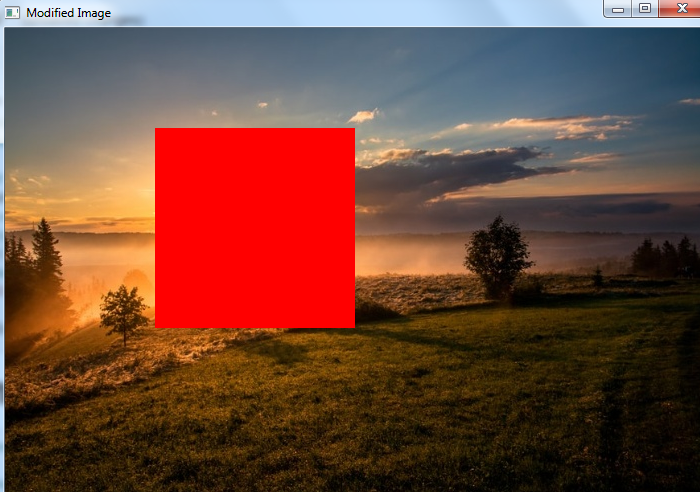# How to access and modify pixel value in an image using OpenCV Python?

To access a single pixel value in an image we can use indexing the same as we do to NumPy array indexing. We can use slicing to access a sequence of pixel values. To modify the pixel values we use the simple Python assignment operator ("=").

## Steps

To access and modify pixel values in an image we could follow the below steps-

• Import the required library. In all the following examples, the required Python library is OpenCV. Make sure you have already installed it.

• Read the input RGB image using cv2.imread(). The RGB image read using this method is in BGR format. Optionally assign the read BGR image to img.

• To access a single pixel use indexing and to modify a single pixel value use assignment to indexing. Like to modify the pixel value at [200,150] to red color we apply

img[200,150] = (0, 0, 255)

• To access a sequence of pixels use slicing and to modify these pixel values use assignment to slicing. Like to modify the pixel values at [100:300,150:350] to red color we apply

img[100:300,150:350] = (0, 0, 255)


We will use this image as the Input File in the following example.## Example

In this Python program, we access the pixel value at a point in the input image. We also find the pixel value for three different color channels and modify the pixel value at that point for the red color channel.

# program to access and modify a pixel value

# import required libraries
import cv2

# access pixel values using indexing
print("pixel value at [200,150]:", img[200,150])
print("pixel value blue channel at [200,150]:", img[200,150])
print("pixel value green channel at [200,150]:", img[200,150])
print("pixel value red channel at[200,150]:", img[200,150])

# modify the pixel value at [200,150] for red color channel
img[200,150] = (0, 0, 255)
print("after modifying pixel value at [200,150]:", img[200,150])


## Output

When you run the above program, it will produce the following output −

pixel value at [200,150]: [115 192 254]
pixel value blue channel at [200,150]: 115
pixel value green channel at [200,150]: 192
pixel value red channel at [200,150]: 254
after modifying pixel value at [200,150]: [ 0 0 255]


## Example

In this Python program, we access the pixel values of a region in the input image. We also modify these pixel values to red color.

# program to access and modify the pixel values of a region

# import required libraries
import cv2

# access pixel values using indexing and slicing

# modify pixel color of a region to red color
img[100:300,150:350] = (0, 0, 255)

# display the modified image
cv2.imshow('Modified Image', img)
cv2.waitKey(0)
cv2.destroyAllWindows()


## Output

When you run the above program, it will produce the following output −Notice the output image after modifying the pixel values to red color.